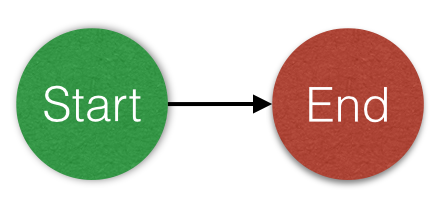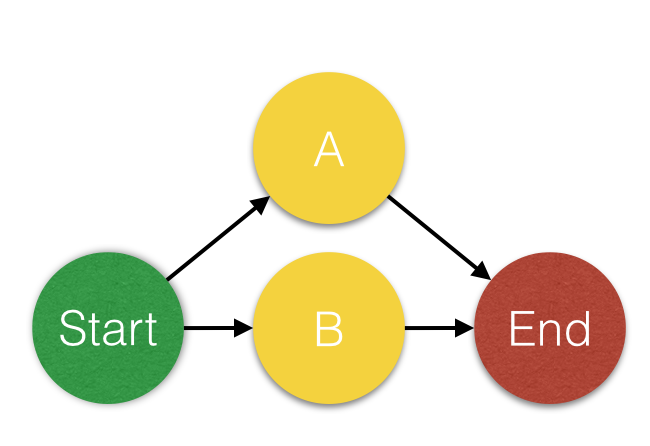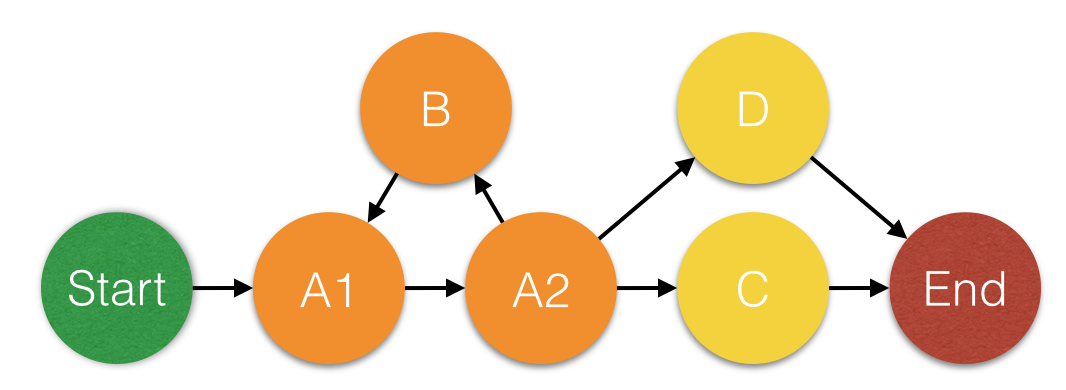# Cyclomatic Complexity

Imagine you're having a great day at the office, you've got a brand new feature the customer is dying to have, and you've completed it in record time. You're all ready to get it merged and peer reviewed.

So you commit it, and push it to your build pipeline, where it's analyzed. The build fails. Your analytics tool tells you complexity is too high!

You're given a numeric complexity value for your code, and a lower complexity value that you need to get your code's complexity under. But what does that exactly does this value mean? And why does high complexity mean you probably need to refactor your code?

Complexity in software development is Cyclomatic Complexity. Cyclomatic Complexity was first described way back in 1976 by Thomas McCabe, and is in it's essence a numeric value that is the total number of paths an execution through a piece of code could take. The higher this number, the higher the number of routes through a piece of code, and the more behaviors your class will exhibit that you'll need to test for (and opportunities your code has to choose the wrong path).

### How to we work out the number?

Take for example this simple method.

``````def hello
puts "hello, world!";
end
``````

We can represent this using a graph. Using a node to represent the start and the end of the code, and a node for every decision made within the graph. In our example there are none, making the complexity simple to work out.Now we've built our graph, we apply this formula defined by McCabe that counts the number of routes through the code.

M = E − N + 2P

What do these letters mean though?

• E = The number of edges of the graph. Edges are the lines in the graph
• N = The number of nodes of the graph. One of the circles is a node
• P = The number of connected components. Number of interchangeable components, like classes, we're analysing

So in this example

• E = 1 There's 1 line in the graph
• N = 2 There's 2 nodes in the graph, Start and End
• P = 1 There's a single component we are analyzing, our simple hello world function

So our formula breaks down like this

1. 1 = 1 - 2 + (2 * 1)
2. 1 = 1 - 2 + 2
3. 1 = -1 + 2
4. 1 = 1

So this method has a complexity of 1. There is 1 route through the code.

Okay, but what if we start making our method more complex? Lets add in an if statement in there.

``````def hello(name=nil)
if name.nil?
puts "hello, world!"; # A
else
puts "hello, "+name+"!"; # B
end
end
``````

We can represent this with a graph like thisSo lets take our formula again

M = E − N + 2P

• E = 4 There's 4 edges in the graph
• N = 4 There's 4 nodes in the graph, Start and End and our if name selection
• P = 1 There's a single component we are analyzing, our simple hello world function

So our formula breaks down like this

1. 2 = 4 - 4 + (2 * 1)
2. 2 = 4 - 4 + 2
3. 2 = 0 + 2
4. 2 = 2

There are 2 routes through the code.

Programs don't just need selection though, they need iteration too! Also known as: Loops. How do we calculate the complexity of this function with a loop in the method?

Here's our updated example with a small loop in it

``````def hello(loops, name=nil)

loops.times do # A1
puts "hello,"; # B
end # A2

if name.nil?
puts "hello, world!"; # C
else
puts "hello, "+name+"!"; # D
end
end
``````

Now representing loops is a little bit more complicated than if statementsYou'll notice that for the loop there are 3 nodes. A1 is the path to the loop, B is the code in the loop, and A2 is the code from the loop till the next statement.

So lets apply our formula again.

M = E − N + 2P

• E = 8
• N = 7
• P = 1

Which means the complexity is

1. 3 = 8 - 7 + (2 * 1)
2. 3 = 8 - 7 + 2
3. 3 = 1 + 2
4. 3 = 3

There are 3 paths through the code.

### But why is this number important?

This number is important because there are 3 main types of bugs in software:

1. Logical If 2 > 3 when it should be if 3 > 4
2. Wiring I injected the queue object when I should have injected the db object
3. Rendering I put the wrong text in this button

Logical is the most common, Wiring the second most common and Rendering the least common. Logical is also the hardest to identify and resolve, Wiring the second most, and Rendering the least.

The complexity number indicates the number of opportunities there are to create a logical error. The lower the complexity, the higher the probability we haven't got a bug in our code.

This is also part of why SOLID encourages you to never have to go back and change a class. Assuming you're adding new functionality to a class, every time you modify it, you're increasing the complexity of the class, which increases the difficulty of testing it, and increases the likelihood you've made a mistake in the logic.

That's what complexity means, and reducing it in your classes and methods will reduct the amount of bugs in your code. Despite it being created a long time ago, it's still an incredibly useful metric.